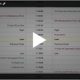# Discover Options Volatility

Options Education Homepage

Understanding Options Volatility

Volatility is the bounciness of the underlying asset of an option.

There are complicated formulas for measuring realized volatility, there are complicated formulas for forecasting volatility, and there are also complicated formulas for calculating implied volatility.

Temperature Change Example

For example, we will look at temperature changes that may occur in different parts of the world. In Singapore, the temperature swings over the course of a year only vary by 15 degrees from the coldest temperature to the hottest. In Bismarck, North Dakota, those same temperatures swings can be as much as 80 degrees. Thus, the temperature volatility is much greater in Bismarck than in Singapore.Asset Class Example

You can also compare the bounciness of natural gas prices to corn prices.

If we look at price changes in percentage terms for natural gas versus corn, we see the natural gas price change, whether up or down, is larger than the corn price change. Therefore, natural gas is bouncier than corn.

Volatility as Measure of Bounciness

Volatility as a measure of bounciness, is simply a standard deviation of the underlying asset.

In the options world, volatility is quoted as an annualized number. You can calculate a one year, one standard deviation move,by taking the volatility times the underlying price.

For example, if the underlying price was 100 and volatility was 20%, a one standard deviation move would be 20 points, up or down. This would create an expected price range of 80 to 120.

Time Horizons

If you have a different time horizon, we can calculate that as well by adjusting the volatility by using the square root of time. For a one-month period, the standard deviation would be 20% times the square root of 1/12. The square root of 1/12 is 0.289. Therefore, the range from an initial price of 100 would be 94.2 to 105.8 in one month. For a one-week period, the standard deviation would be 20% times the square root of 1/52. A one standard deviation range for a week, would be 97.2 to 102.8.Summary

The bounciness of an asset is referred to as volatility, which is the standard deviation. In the options world, that standard deviation is always annualized. The standard deviation can be scaled to different time periods.

We have demonstrated how you could compare the bounciness, or volatility, of different underlying assets by annualizing the standard deviations of those assets.

If you have questions send us a message or schedule an online review .

Regards,

—————————————————————-

Privacy Notice

Disclosure